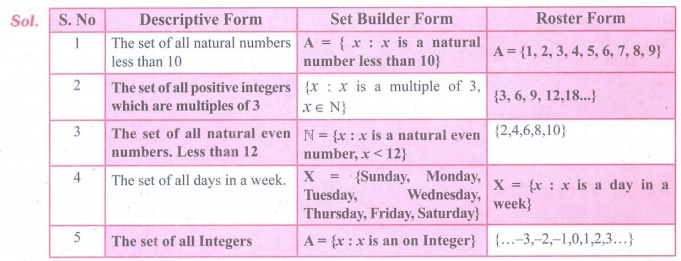Home | | Maths 9th std | Representation of a Set

# Representation of a Set

A set can be represented in any one of the following three ways or forms: 1) Descriptive Form. 2) Set-Builder Form or Rule Form. 3) Roster Form or Tabular Form.

Representation of a Set

The collection of odd numbers can be described in many ways:

1)       â€śThe set of odd numbersâ€ť is a fine description, we understand it well.

2)       It can be written as {1, 3, 5, â€¦} and you know what I mean.

3)       Also, it can be said as the collection of all numbers x where x is an odd number.

All of them are equivalent and useful. For instance,the two descriptions â€śThe collection of all solutions to the equation xâ€“5 = 3â€ť and {8} refer to the same set.

A set can be represented in any one of the following three ways or forms:

1)       Descriptive Form.

2)       Set-Builder Form or Rule Form.

3)       Roster Form or Tabular Form.

## 1. Descriptive Form

In descriptive form, a set is described in words.

For example,

(i) The set of all vowels in English alphabets.

(ii)The set of whole numbers.

## 2. Set Builder Form or Rule Form

In set builder form, all the elements are described by a rule.

For example,

(i) = {: x is a vowel in English alphabets}

(ii) = {x|is a whole number}## 3. Roster Form or Tabular Form

A set can be described by listing all the elements of the set.

For example,

(i) = {a, e, i, o, u}

(ii) = {0,1,2,3,â€¦}

Can this form of representation be possible always?### Example 1.2

Write the set of letters of the following words in Roster form

(i) ASSESSMENT (ii) PRINCIPAL

Solution

(i) ASSESSMENT

A= {A, S, E, M, N, T}

(ii) PRINCIPAL

B={P, R, I, N, C, A, L}Tags : Maths , 9th Maths : UNIT 1 : Set Language
Study Material, Lecturing Notes, Assignment, Reference, Wiki description explanation, brief detail
9th Maths : UNIT 1 : Set Language : Representation of a Set | Maths